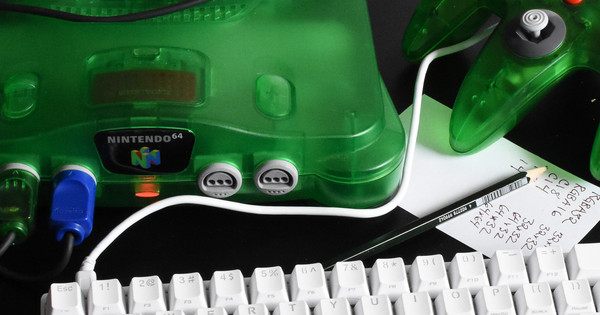# Nintendo 64 Part 24: Quick ’n’ Dirty Collisions

, Nintendo 64, Programming

Very busy with making the game, so I’ll keep this short. It’s time to add collision detection.

Just kidding! I’m using nested for loops and just iterating over all pairs of physics objects. With these kind of time constraints, fancy algorithms don’t make sense. Here is the relevant part of the physics component for entities in the game.

``````// Physical object component. Used for entities with a physical
// presence in the game world.
struct physics_entity {
vec2 pos;      // Position: updated by physics.
vec2 vel;      // Velocity: must be set as an input.
float max_vel; // Maximum velocity after collision response.

// Private.
bool collided; // Has collision adjustment.
bool stable;   // True if component has stable position.
};``````

During update, the first step is moving all of the entities forward.

``````struct physics_entity *ents = ...;
int count = ...;

// Move forwards.
for (int i = 0; i < count; i++) {
// madd(x, y, a) = x + y * a
ents[i].collided = false;
}``````

This may cause some entities to overlap, so the physics system pushes them apart until they don’t overlap any more. The distance each entity is pushed is stored in a field named `adj` which will be used later.

``````// Find collisions between entities and push entities out of
// collisions.
for (int i = 0; i < count; i++) {
for (int j = i + 1; j < count; j++) {
physics_update_pair(&ents[i], &ents[j]);
}
}

// Resolve a collision between a pair of objects, if there is one.
void physics_update_pair(struct physics_entity *px,
struct physics_entity *py) {
vec2 delta_pos = vec2_sub(px->pos, py->pos);
return;
}
float dist2 = vec2_length(delta_pos);
return;
}
float dist = sqrtf(dist2);
float overlap = radius - dist;
if (overlap <= 0.0f) {
return;
}
float adj_amount = 0.5f * overlap / dist;
px->collided = true;
py->collided = true;
bool stable = px->stable & py->stable;
px->stable = stable;
py->stable = stable;
}``````

Once the entities have been pushed out of the way, update their velocity to reflect how much they have been pushed… unless they are not marked as “stable”. The problem here is that if you spawn an entity, it might spawn on top of an existing entity and need to be pushed very far during collision resolution. This would cause the objects to go shooting away from each other at high speed! To fix this, collisions will only affect the velocity of stable objects. An object is unstable when it is created and it becomes unstable again if it collides with an unstable object. At the end of each frame, all objects are marked as stable.

``````// Update the state of entities after collisions.
void physics_post_collision(struct physics_entity *ents, int count,
float invdt) {
for (int i = 0; i < count; i++) {
struct physics_entity *ent = &ents[i];
if (ent->collided) {
if (ent->stable) {
// When an entity pops in, don't accumulate velocity
float maxv2 = ent->max_vel * ent->max_vel;
float v2 = vec2_length2(ent->vel);
if (v2 > maxv2) {
ent->vel = vec2_scale(ent->vel, sqrtf(v2) / ent->max_vel);
}
}
}
}
}

// Update velocity after collision update.
physics_post_collision(cps, psys->count, 1.0f / dt);``````

## Results

The player and various monsters can no longer walk through each other.

Here are the resulting ROM images, available for both NTSC and PAL: